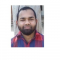## How to write plot description outside plot in facetted plot using ggplot2 in R?Nizamuddin Siddiqui

Updated on 22-Nov-2021 08:14:29

To write plot description outside plot using ggplot2, we can use annotate function and coord_cartesian function. The annotate function will define the plot description and the coord_cartesian function will define the position of the text outside the plot area.Check out the below given example to understand how it works.ExampleFollowing snippet ... Read More

## How to test if strings stored in a vector are present in an R list?Nizamuddin Siddiqui

Updated on 22-Nov-2021 08:11:43

To test if strings stored in a vector are present in an R list or not, we can use mapply function. For example, if we have a vector of strings say V and a list called LIST then we can check whether elements in V are present in LIST by ... Read More

## Find the frequency of successive occurrences less than equal to a threshold in an R data frame column?Nizamuddin Siddiqui

Updated on 22-Nov-2021 08:09:28

To find the frequency of successive occurrences less than a threshold value in an R data frame column, we can use rle function along with sum function.Check out the below given examples to understand how it can be done.Example 1Following snippet creates a sample data frame −x

## How to find numbers that are divisible by a certain number for a range of values in R?Nizamuddin Siddiqui

Updated on 22-Nov-2021 07:53:15

In R, the divisibility of a number by a certain number can be found by using the modulus operator %%. If we want to check the divisibility of a set of numbers by a certain number then for loop will be used.Check out the below given examples to understand how ... Read More

## How to create a scatterplot with white background and no gridlines using ggplot2 in R?Nizamuddin Siddiqui

Updated on 22-Nov-2021 07:45:48

Practically, the scatterplots are well visualized on white background just like on white paper. If we want to create a scatterplot with white background and without gridlines using ggplot2 then we can apply classic theme to the plot.Check out the below given example to understand how it can be done.ExampleFollowing ... Read More

## How to find the number of unique values in comma separated strings stored in an R data frame column?Nizamuddin Siddiqui

Updated on 22-Nov-2021 07:41:06

If we have comma separated values that contains duplicate and unique values then we might want to find the number of unique values within each comma separated value. To find the unique values in comma separated strings stored in an R data frame column, we can use stri_extract_all_regex function of ... Read More

## How to combine columns by excluding missing values in R?Nizamuddin Siddiqui

Updated on 22-Nov-2021 07:34:28

If we have a data set that contains missing values at alternate places for each column then we might want to combine the columns by excluding those missing values, this will reduce the data set and the analysis is likely to become easier.For this purpose, we can use na.exclude function ... Read More

## How to create a colored box for base R plot?Nizamuddin Siddiqui

Updated on 22-Nov-2021 07:29:51

To create a colored box for base R plot, we can use box function with col argument where we can pass the color other than black because black is the default color.We first need to create the plot using plot function and then box function will be used as shown ... Read More

## How to create a matrix in R by filling the data with predefined values in loop?Nizamuddin Siddiqui

Updated on 22-Nov-2021 07:25:17

If we know the total number of rows we want in our matrix and the number of columns then we can use matrix function to create a matrix by filling the data with predefined values. These values must be equal to the multiplication of number of rows and columns.Check out ... Read More

## How to create ACF plot in R?Nizamuddin Siddiqui

Updated on 22-Nov-2021 07:22:14

The autocorrelation plot or ACF plot is a display of serial correlation in data that changes over time. The ACF plot can be easily created by using acf function.For example, if we have a vector called V then we can create its autocorrelation plot by using the command given below ... Read More